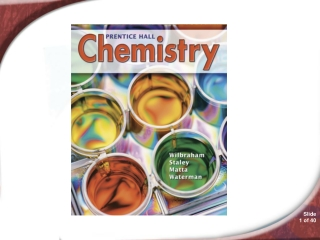DownloadDownload Presentationchemistry

# chemistry

Télécharger la présentation## chemistry

- - - - - - - - - - - - - - - - - - - - - - - - - - - E N D - - - - - - - - - - - - - - - - - - - - - - - - - - -
##### Presentation Transcript

1. chemistry

2. 10.3 Percent Composition and Chemical Formulas • It helps to know the percents of the components in a shirt because they affect how warm it is, whether it will need to be ironed, and how it should be cleaned. You will learn how the percents of the elements in a compound are important in chemistry.

3. 10.3 The Percent Composition of a Compound • The Percent Composition of a Compound • How do you calculate the percent by mass of an element in a compound?

4. 10.3 The Percent Composition of a Compound • The percent by mass of an element in a compound is the number of grams of the element divided by the mass in grams of the compound, multiplied by 100%.

5. 10.3 The Percent Composition of a Compound • Percent Composition from Mass Data • The relative amounts of the elements in a compound are expressed as the percent composition or the percent by mass of each element in the compound.

6. 10.9

7. 10.9

8. 10.9

9. 10.9

10. for Sample Problem 10.9 Problem Solving 10.33 Solve Problem 33 with the help of an interactive guided tutorial.

11. 10.3 The Percent Composition of a Compound • Percent Composition from the Chemical Formula

12. 10.10

13. 10.10

14. 10.10

15. 10.10

16. for Sample Problem 10.10 Problem Solving 10.34 Solve Problem 34 with the help of an interactive guided tutorial.

17. 10.3 The Percent Composition of a Compound • Percent Composition as a Conversion Factor • You can use percent composition to calculate the number of grams of any element in a specific mass of a compound.

18. 10.3 The Percent Composition of a Compound • Propane (C3H8) is 81.8% carbon and 18% hydrogen. You can calculate the mass of carbon and the mass of hydrogen in an 82.0 g sample of C3H8.

19. 10.3 Empirical Formulas • Empirical Formulas • What does the empirical formula of a compound show?

20. 10.3 Empirical Formulas • The empirical formula gives the lowest whole-number ratio of the atoms of the elements in a compound. • The empirical formula of a compound shows the smallest whole-number ratio of the atoms in the compound.

21. 10.3 Empirical Formulas • Ethyne (C2H2) is a gas used in welder’s torches. Styrene (C8H8) is used in making polystyrene. • These two compounds of carbon have the same empirical formula (CH) but different molecular formulas.

22. 10.11

23. 10.11

24. 10.11

25. 10.11

26. for Sample Problem 10.11 Problem-Solving 10.37 Solve Problem 37 with the help of an interactive guided tutorial.

27. 10.3 Molecular Formulas • Molecular Formulas • How does the molecular formula of a compound compare with the empirical formula?

28. 10.3 Molecular Formulas • The molecular formula of a compound is either the same as its experimentally determined empirical formula, or it is a simple whole-number multiple of its empirical formula.

29. 10.3 Molecular Formulas Methanal, ethanoic acid, and glucose all have the same empirical formula—CH2O.

30. 10.3 Molecular Formulas

31. 10.12

32. 10.12

33. 10.12

34. 10.12

35. for Sample Problem 10.12 Problem-Solving 10.38 Solve Problem 38 with the help of an interactive guided tutorial.

36. 10.3 Section Quiz. • 10.3.

37. 10.3 Section Quiz. • 1. Calculate the percent by mass of carbon in cadaverine, C5H14N2, a compound present in rotting meat. • 67.4% C • 58.8% C • 51.7% C • 68.2% C

38. 10.3 Section Quiz. • 2. Which of the following is NOT an empirical formula? • NO2 • H2N • CH • C3H6

39. 10.3 Section Quiz. • 3. Determine the molecular formula of a compound that contains 40.0 percent C, 6.71 percent H, and 53.29 percent O and has a molar mass of 60.05 g. • C2H4O2 • CH2O • C2H3O • C2H4O

40. Concept Map 10 Concept Map 10 Solve the Concept Map with the help of an interactive tutorial.

41. END OF SHOW